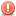##how show rray in multi textbox

hi
I wrote this code, but I do not know why the text box only shows an array
I want all arrays in a matrix display

object[,] matrix = new object[3, 3];

for (int i = 0; i < 2; i++)
{
for (int j = 0; j < 2; j++)
{
matrix[i, j] = 5;
textBox4.Text= matrix[i,j].ToString();

}
}

}